# 【模板应用】JumpServer 安全运维审计大屏的设计与实现

0 评论 / 0 点赞 / 536 阅读 最后更新: 2022-09-20 作者: 飞致云 总字数: 4013

# 1 前言

2022年6月21日，DataEase 开源数据库可视化分析平台正式发布模板市场。其中作为众多模版之一的 JumpServer 安全运维审计大屏之一，备受大家青睐。应广大用户要求，在此详细讲解如何应用安全运维审计大屏模版。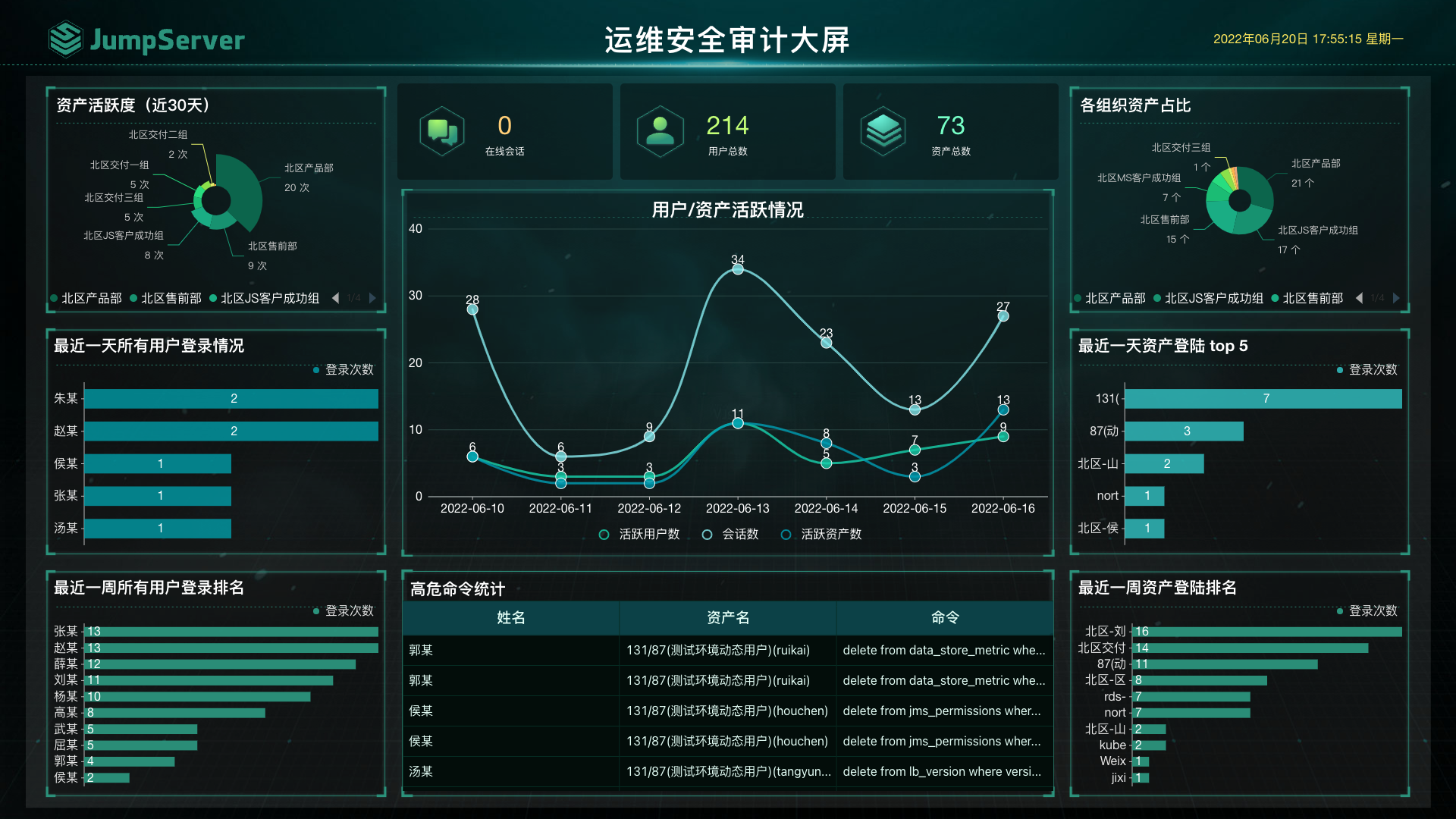# 2 大屏场景

## 2.2 统计指标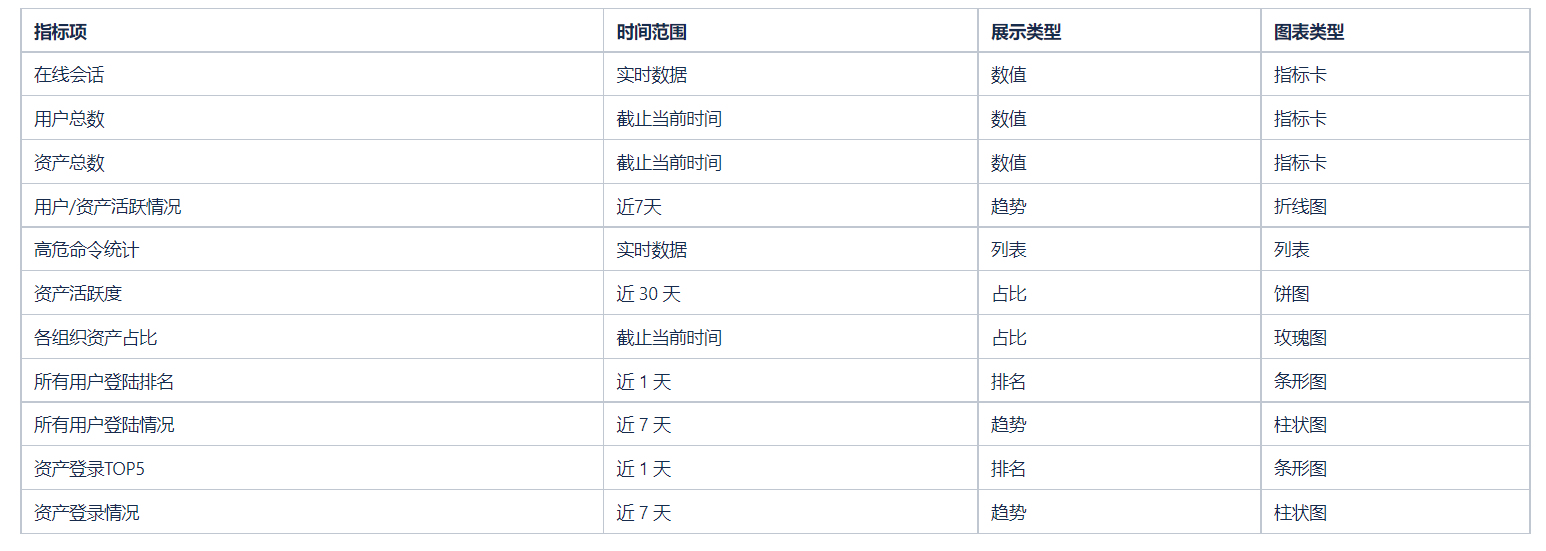# 3 模版应用

## 3.1 模版下载

https://dataease.io/templates/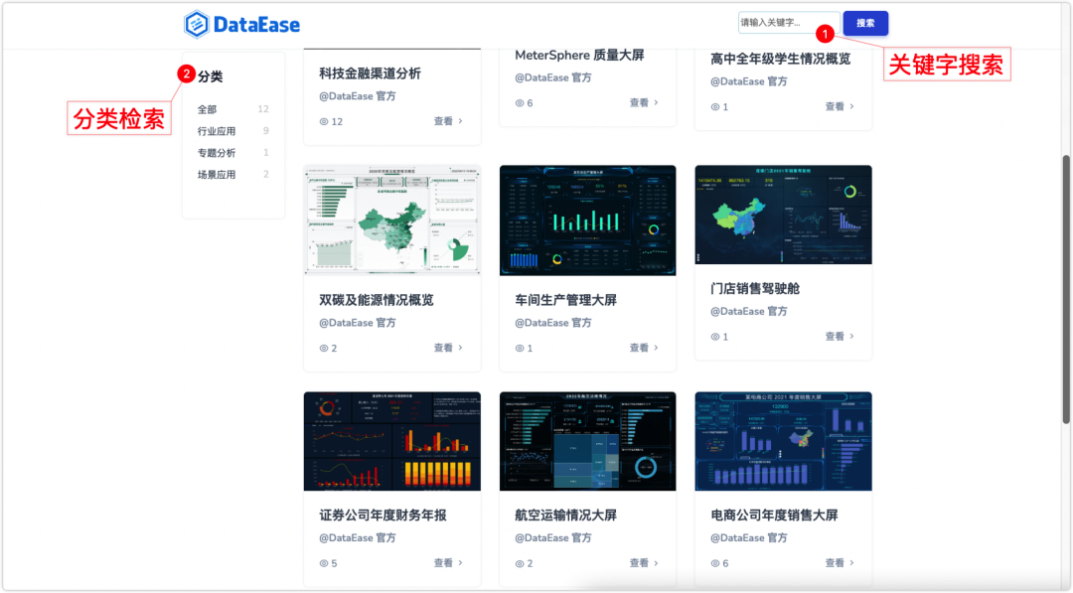## 3.2 模版导入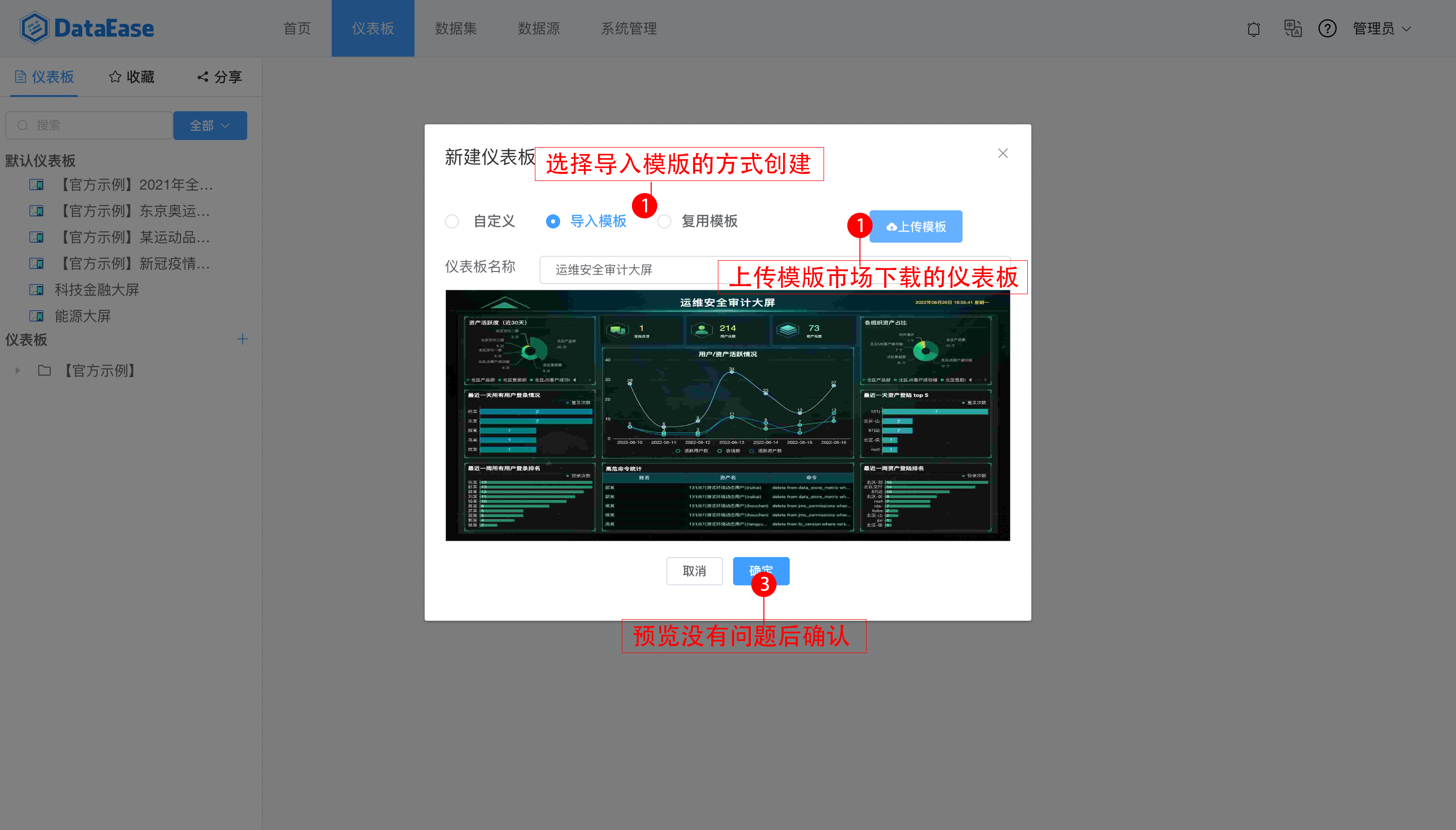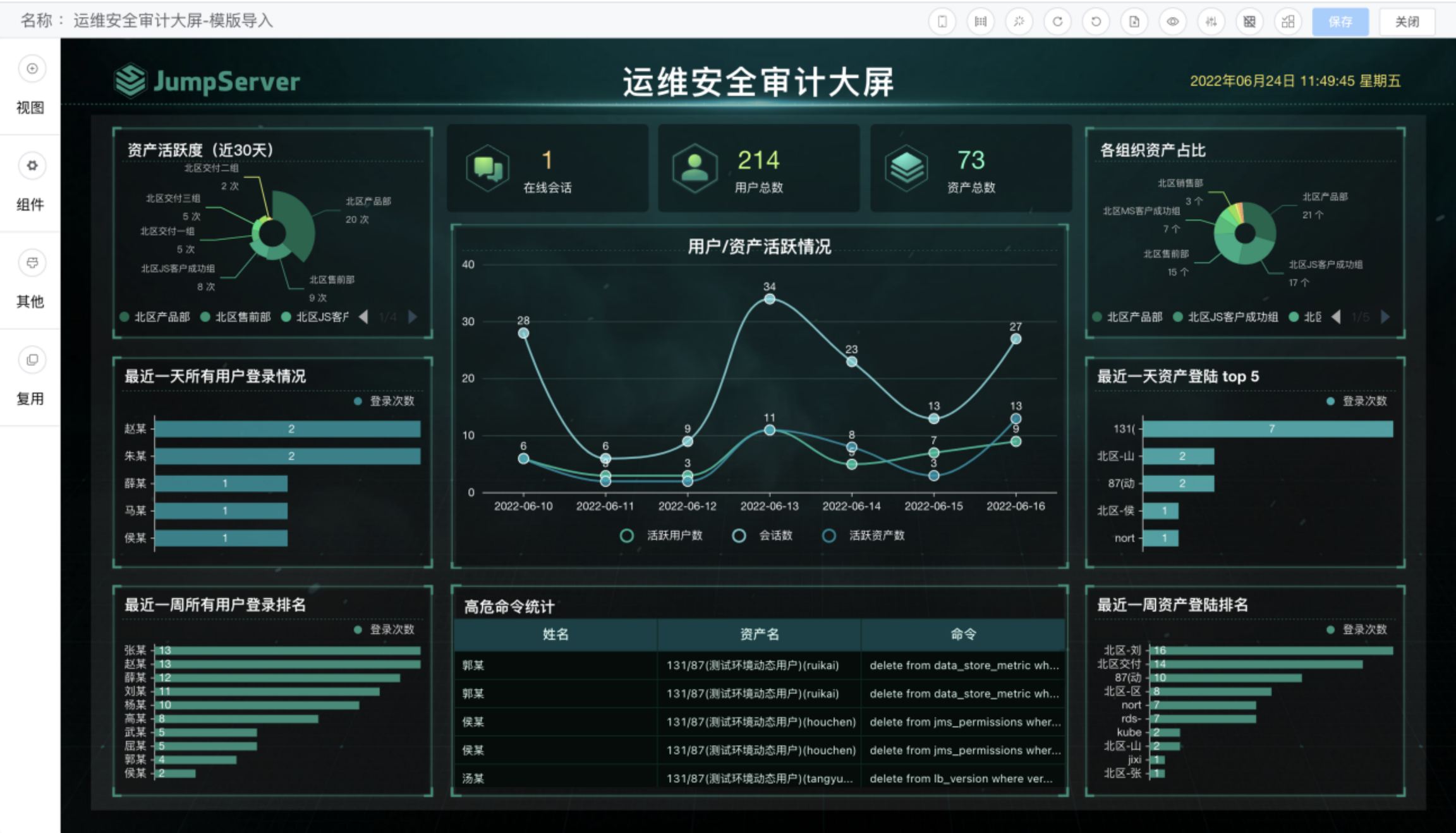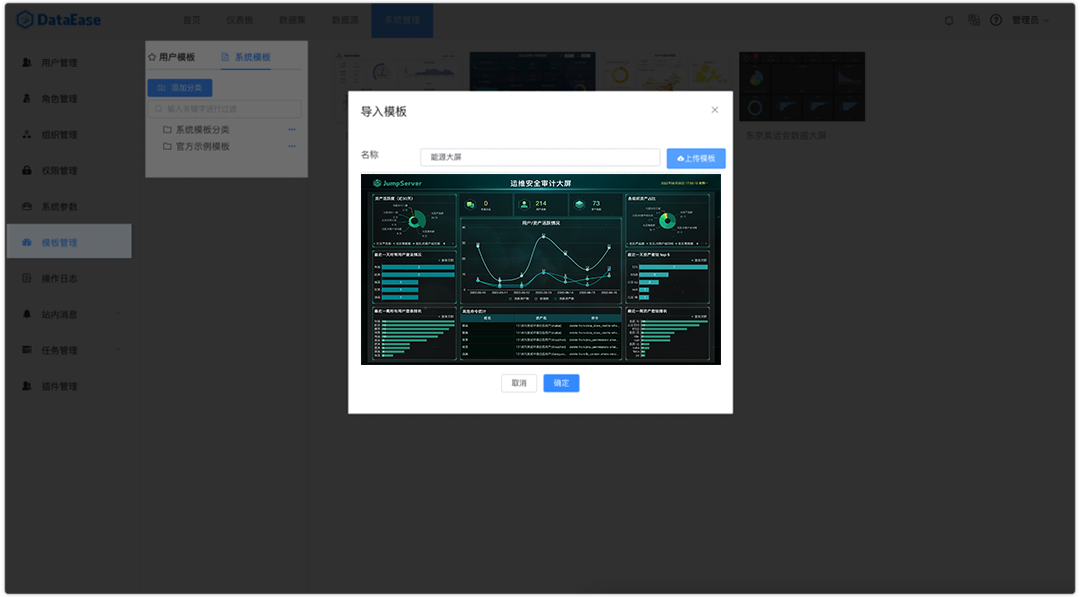## 3.3 接入数据源

DataEase 最新版本支持多种数据库作为数据源，JumpServer 使用的是 MySQL 数据库，这里输入相应信息，创建数据源。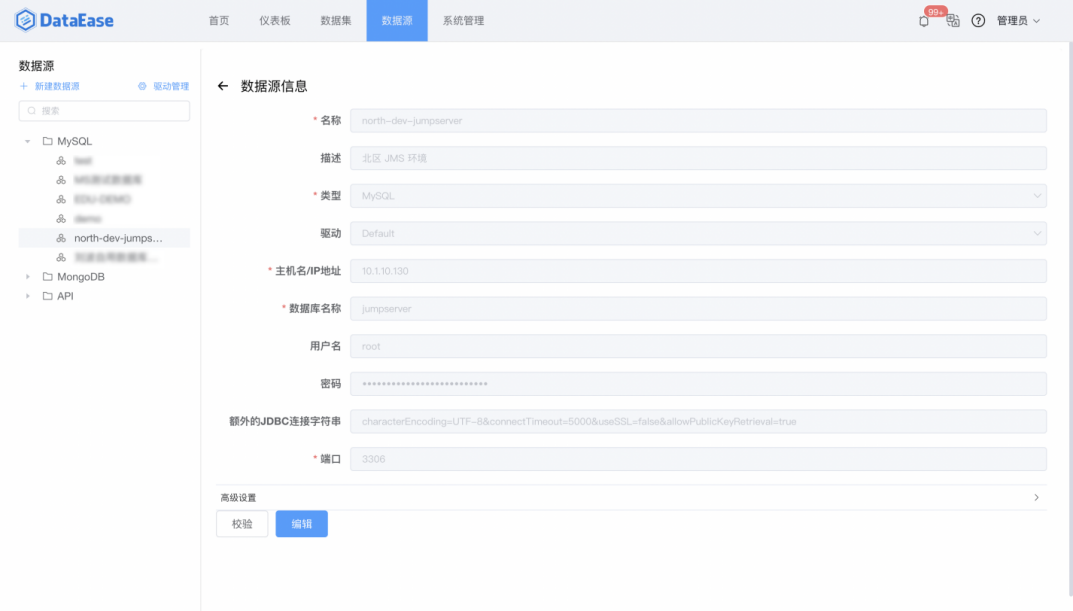## 3.4 添加数据集

https://dataease.io/docs/user_manual/dataset_configuration/dataset_SQL/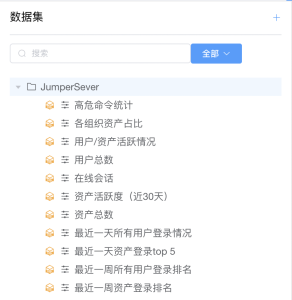# 4 脚本语句

## 4.1 在线会话

1. 指标说明
查询当前正在进行中的会话数量。

2. SQL 语句

``````在线会话
select count(1) '在线会话数' from terminal_session
where is_finished = false
``````

## 4.2 用户总数

1. 指标说明
查询用户总数。

2. SQL 语句

``````用户总数
select count(*) '用户总数' from users_user where role <> 'App'
``````

## 4.3 资产总数

1. 指标说明
查询所有组织资源数量。

2. SQL 语句

``````资产总数
select count(1) '资产总数' from assets_asset
``````

## 4.4 用户/资产活跃情况

1. 指标说明
查询近期用户活跃情况、资产活跃情况、历史在线会话情况。

2. SQL 语句

``````资产总数
select u.`日期`,u.`活跃用户数`,a.`活跃资产数`,s.`会话数` from (select count(distinct user_id) '活跃用户数', d '日期' from (select DATE_FORMAT(date_start, '%Y-%m-%d') d, user_id from terminal_session where date_start > DATE_SUB(CURDATE(), INTERVAL 7 DAY))t group by d ORDER BY d DESC LIMIT 7) u
left join (select count(distinct asset_id) '活跃资产数', d '日期' from (select DATE_FORMAT(date_start, '%Y-%m-%d') d, asset_id from terminal_session where date_start > DATE_SUB(CURDATE(), INTERVAL 7 DAY))t group by d ORDER BY d DESC LIMIT 7) a
on u.`日期` = a.`日期`
left join (select d '日期', count(1) '会话数' from ( select DATE_FORMAT(date_start, '%Y-%m-%d') d from terminal_session where date_start > DATE_SUB(CURDATE(), INTERVAL 7 DAY)) t group by d ORDER BY d DESC LIMIT 7) s
on u.`日期` = s.`日期`
``````

## 4.5 高危命令统计

1. 指标说明
查询高危命令情况。

2. SQL 语句

``````高危命令统计
SELECT * FROM terminal_command WHERE risk_level !='0'
``````

## 4.6 资产活跃度（近 30 天）

1. 指标说明
查询近 30 天的资产活跃度。

2. SQL 语句

``````资产活跃度（近30天）
select o.name '组织' , count(count_num ) '活跃度'
from orgs_organization o
join
(SELECT
asset, count(*) count_num,
REPLACE(org_id,'-','') org_id
FROM
terminal_session t
WHERE
t.is_finished = 1
and t.protocol='ssh'
AND datediff( NOW(), t.date_end ) <= 30
GROUP BY
org_id, asset) terminal on o.id=terminal.org_id
group by o.name
``````

## 4.7 各组织资产占比

1. 指标说明
查询所有组织服务器数量。

2. SQL 语句

``````各组织资产占比
SELECT t.NAME, COUNT( * ) AS 服务器数量
FROM assets_asset a, orgs_organization t
WHERE LEFT ( a.org_id, 8 ) = LEFT ( t.id, 8 )
GROUP BY t.NAME ORDER BY 服务器数量 DESC
``````

## 4.8 最近一天所有用户登录情况

1. 指标说明
查询最近一天所有用户登录次数。

2. SQL 语句

``````最近一天所有用户登录情况
select name 用户名,login_time 登录次数 from (
SELECT
uu.name name,
count( * ) AS login_time
FROM
WHERE
TO_DAYS( now( ) ) - TO_DAYS( datetime ) <= 1
GROUP BY
)t
where name is not null
ORDER BY
``````

## 4.9 最近一周所有用户登录排名

1. 指标说明
查询最近一周所有用户登录次数

2. SQL 语句

``````最近一周所有用户登录排名
SELECT
(select name from users_user uu where uu.username = au.username) as name,
count( * ) AS 登录次数
FROM
WHERE
TO_DAYS( now( ) ) - TO_DAYS( datetime ) <= 7
GROUP BY
ORDER BY

``````

## 4.10 最近一天资产登录 top 5

1. 指标说明
查询最近一天资产的被访问次数。

2. SQL 语句

``````最近一天资产登录top 5
SELECT
asset,
assets_asset.ip,
COUNT( * ) AS 登录次数
FROM
terminal_session
left join assets_asset
on replace(terminal_session.asset_id, '-', '') = assets_asset.id
WHERE
TO_DAYS( now( ) ) - TO_DAYS( date_end ) <= 1
GROUP BY
asset
ORDER BY

``````

## 4.11 最近一周资产登录排名

1. 指标说明
查询最近一周所有资产的登录次数。

2. SQL 语句

``````最近一周资产登录排名
SELECT
asset,
assets_asset.ip as ip,
COUNT( * ) AS 登录次数
FROM
terminal_session
left join assets_asset
on replace(terminal_session.asset_id, '-', '') = assets_asset.id
WHERE
TO_DAYS( now( ) ) - TO_DAYS( date_end ) <= 7
GROUP BY
asset
ORDER BY

``````

# 5 数据替换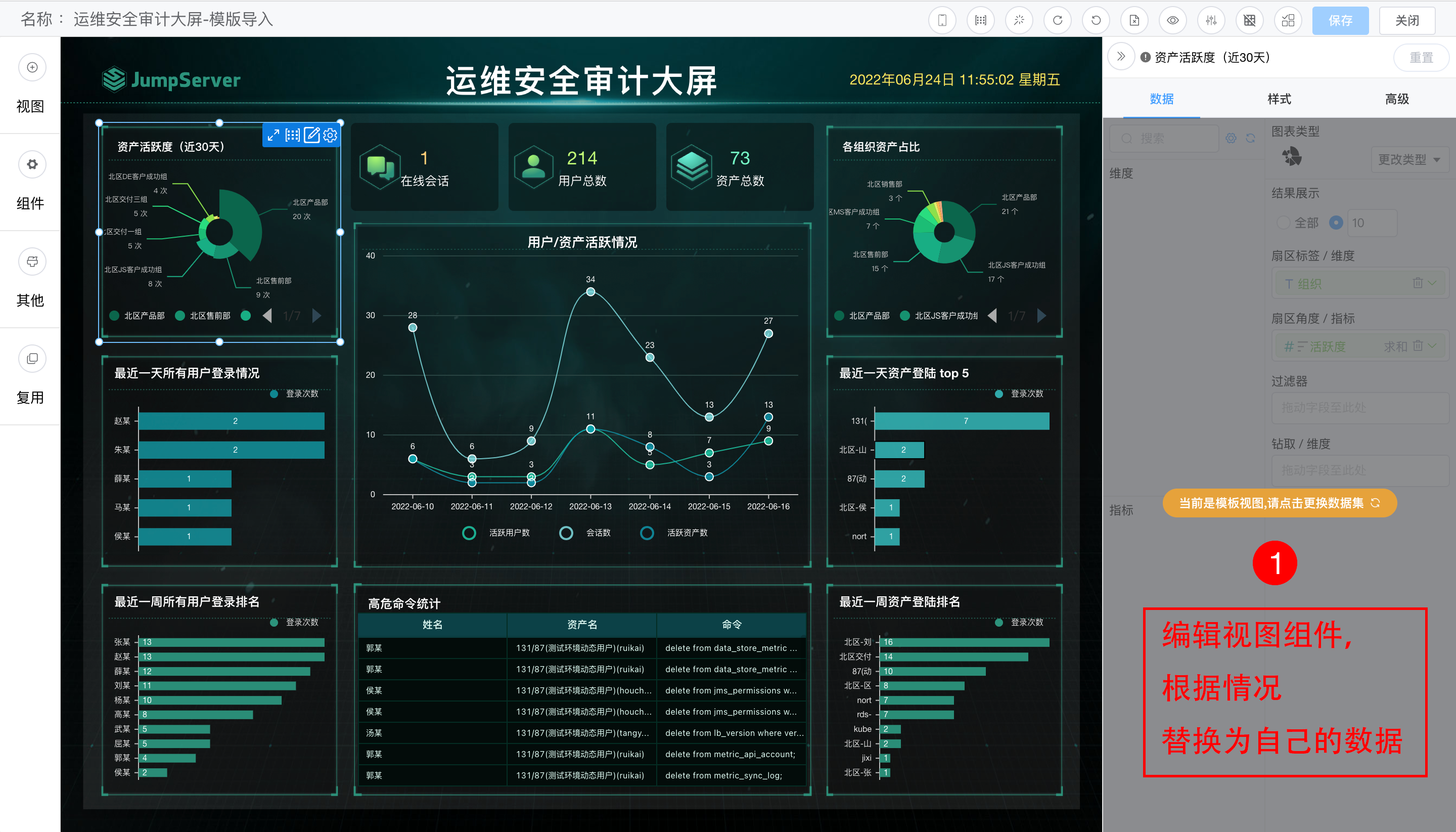1. 类别轴/维度字段缺失
由于在模版设计阶段涉及到了人员名称等敏感信息，因此在数据集内新建了脱敏字段，因此在替换数据集时在数据维度区域会出现下图红框所示情况。再此，大家可以将红框指标删除，把“用户名”字段直接拖入所示位置即可。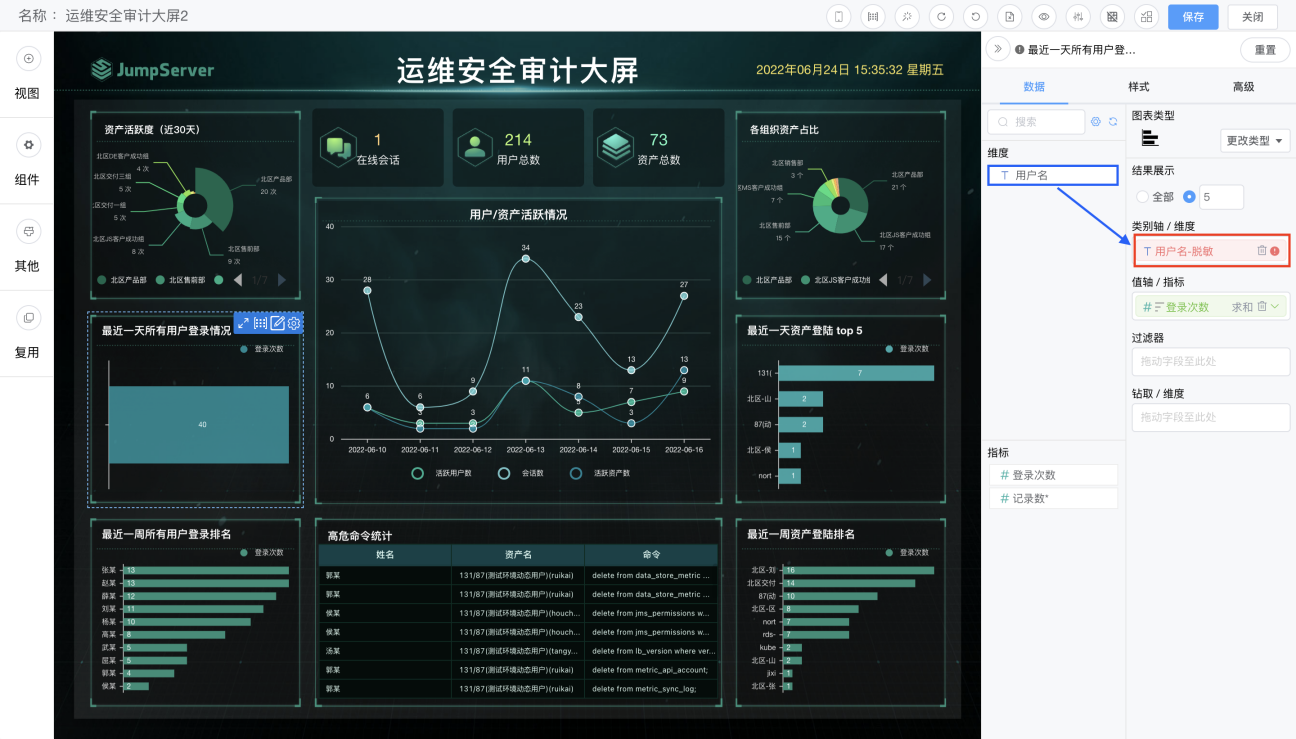2. 替换字段后维度字段过长图表显示不全。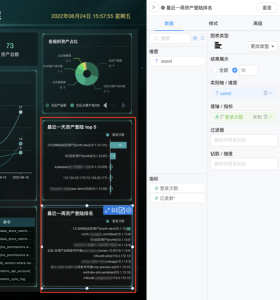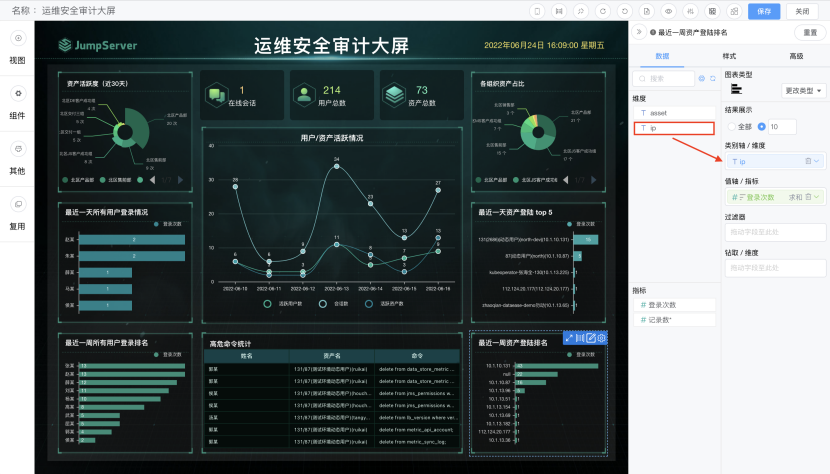# 6 最终效果